# A Comprehensive Guide To Artificial Intelligence With Python

## Artificial Intelligence With Python

Artificial Intelligence has been around for over half a century now and its advancements are growing at an exponential rate. The demand for AI is at its peak and if you wish to learn about Artificial Intelligence, you’ve landed at the right place. This blog on Artificial Intelligence With Python will help you understand all the concepts of AI with practical implementations in Python.

To get in-depth knowledge of Artificial Intelligence and Machine Learning, you can enroll for live Machine Learning Engineer Master Program by Edureka with 24/7 support and lifetime access.

The following topics are covered in this Artificial Intelligence With Python blog:

1. Why Is Python Best For AI?
2. Demand For AI
3. What Is Artificial Intelligence?
4. Types Of Artificial Intelligence
5. Machine Learning Basics
6. Types Of Machine Learning
7. Types Of Problems Solved By Using Machine Learning
8. Machine Learning Process
9. Machine Learning With Python
10. Limitations Of Machine Learning
11. Why Deep Learning?
12. How Deep Learning Works?
13. What Is Deep Learning?
14. Deep Learning Use Case
15. Perceptrons
16. Multilayer Perceptrons
17. Deep Learning With Python
18. Introduction To Natural Language Processing (NLP)
19. NLP Applications
20. Terminologies In NLP

## Why Is Python Best For AI?

A lot of people have asked me, ‘Which programming language is best for AI?’ or “Why Python for AI?”

Despite being a general purpose language, Python has made its way into the most complex technologies such as Artificial Intelligence, Machine Learning, Deep Learning, and so on.

Why has Python gained so much popularity in all these fields?

Here is a list of reasons why Python is the choice of language for every core Developer, Data Scientist, Machine Learning Engineer, etc:Why Python For AI – Artificial Intelligence With Python – Edureka

• Less Code: Implementing AI involves tons and tons of algorithms. Thanks to Pythons support for pre-defined packages, we don’t have to code algorithms. And to make things easier, Python provides “check as you code” methodology that reduces the burden of testing the code.
• Prebuilt Libraries: Python has 100s of pre-built libraries to implement various Machine Learning and Deep Learning algorithms. So every time you want to run an algorithm on a data set, all you have to do is install and load the necessary packages with a single command. Examples of pre-built libraries include NumPy, Keras, Tensorflow, Pytorch, and so on.
• Ease of learning: Python uses a very simple syntax that can be used to implement simple computations like, the addition of two strings to complex processes such as building a Machine Learning model.
• Platform Independent: Python can run on multiple platforms including Windows, MacOS, Linux, Unix, and so on. While transferring code from one platform to the other you can make use of packages such as PyInstaller that will take care of any dependency issues.
• Massive Community Support: Python has a huge community of users which is always helpful when we encounter coding errors. Apart from a huge fan following, Python has multiple communities, groups, and forums where programmers post their errors and help each other.

If you wish to learn Python Programming in depth, here are a couple of links, do give these blogs a read:

1. Python Tutorial – A Complete Guide to Learn Python Programming
3. A Beginners Guide To Python Functions
4. Python for Data Science

Since this blog is all about Artificial Intelligence With Python, I will introduce you to the most effective and popular AI-based Python Libraries.

1. Tensorflow: Developed by Google, this library is popularly used in writing Machine Learning algorithms and performing heavy computations that involve Neural Networks.
2. Scikit-Learn: Scikit-learn is a Python library associated with NumPy and SciPy. It is considered as one of the best libraries for working with complex data.
3. NumPy: Numpy is a python library specifically used for computing scientific/mathematical data.
4. Theano: Theano is a functional library that effectively calculates and computes mathematical expressions involving multi-dimensional arrays.
5. Keras: This library simplifies the implementation of neural networks. It also has the best functionalities for computing models, evaluating data-sets, visualizing graphs, and much more.
6. NLTK: NLTK or Natural Language ToolKit is an open source Python library specifically built for Natural Language Processing, text analysis, and text mining.

In addition to the above-mentioned libraries make sure you check out this Top 10 Python Libraries You Must Know In 2019 blog to get a more clear understanding.

Now that you know the important Python libraries that are used for implementing AI techniques, let’s focus on Artificial Intelligence. In the next section, I will cover all the fundamental concepts of AI.

First, let’s start by understanding the sudden demand for AI.

## Demand For AI

Since the emergence of AI in the 1950s, we have seen exponential growth in it’s potential. But if AI has been here for over half a century, why has it suddenly gained so much importance? Why are we talking about Artificial Intelligence now?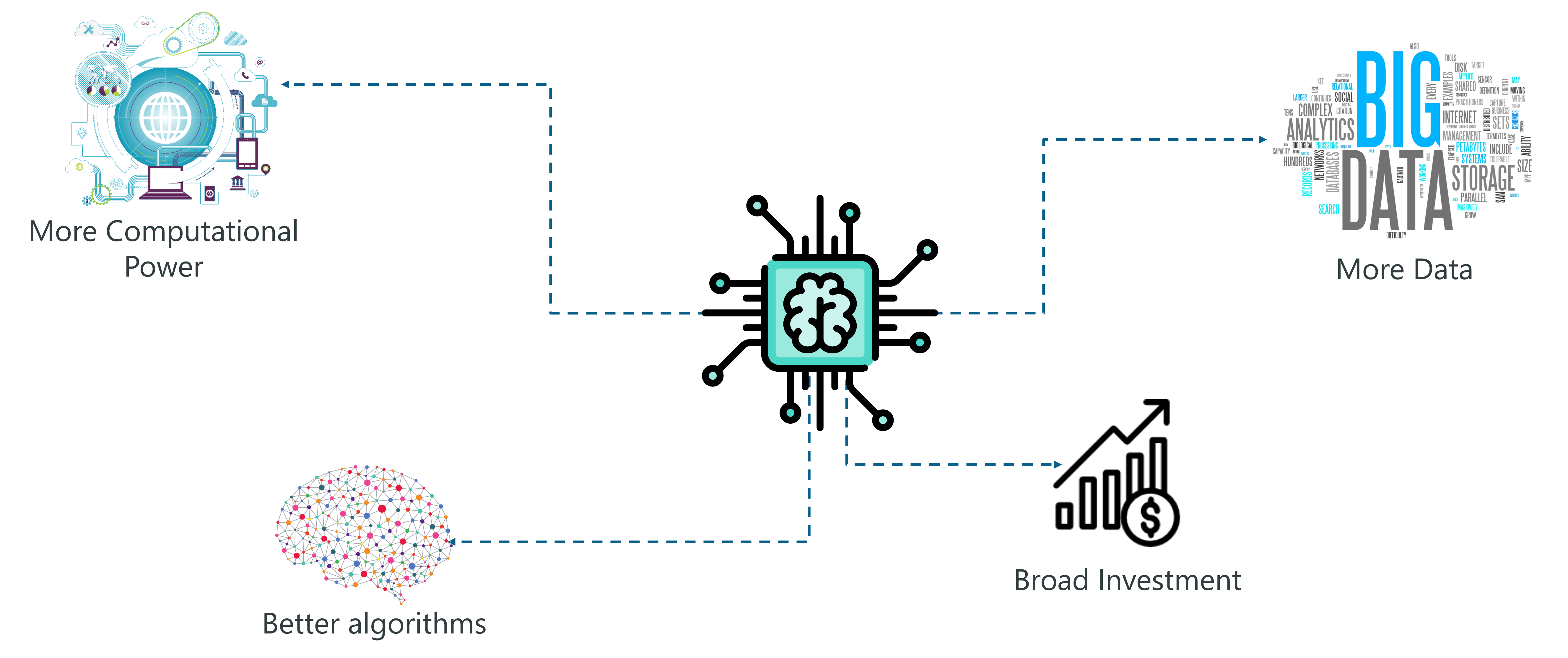Demand For AI – Artificial Intelligence With Python – Edureka

The main reasons for the vast popularity of AI are:

More computing power: Implementing AI requires a lot of computing power since building AI models involve heavy computations and the use of complex neural networks. The invention of GPUs has made this possible. We can finally perform high-level computations and implement complex algorithms.

Data Generation: Over the past years, we’ve been generating an immeasurable amount of data. Such data needs to be analyzed and processed by using Machine Learning algorithms and other AI techniques.

More Effective Algorithms: In the past decade we’ve successfully managed to develop state of the art algorithms that involve the implementation of Deep Neural Networks.

Broad Investment: As tech giants such as Tesla, Netflix and Facebook started investing in Artificial Intelligence, it gained more popularity which led to an increase in the demand for AI-based systems.

The growth of Artificial Intelligence is exponential, it is also adding to the economy at an accelerated pace. So this is the right time for you to get into the field of Artificial Intelligence.

Check out these AI and Machine Learning courses by E & ICT Academy NIT Warangal to learn and build a career in Artificial Intelligence.

## What Is Artificial Intelligence?

The term Artificial Intelligence was first coined decades ago in the year 1956 by John McCarthy at the Dartmouth conference. He defined AI as:

“The science and engineering of making intelligent machines.”

In other words, Artificial Intelligence is the science of getting machines to think and make decisions like humans.

In the recent past, AI has been able to accomplish this by creating machines and robots that have been used in a wide range of fields including healthcare, robotics, marketing, business analytics and many more.

Now let’s discuss the different stages of Artificial Intelligence.

## Types Of Artificial Intelligence

AI is structured along three evolutionary stages:

1. Artificial Narrow Intelligence
2. Artificial General Intelligence
3. Artificial Super Intelligence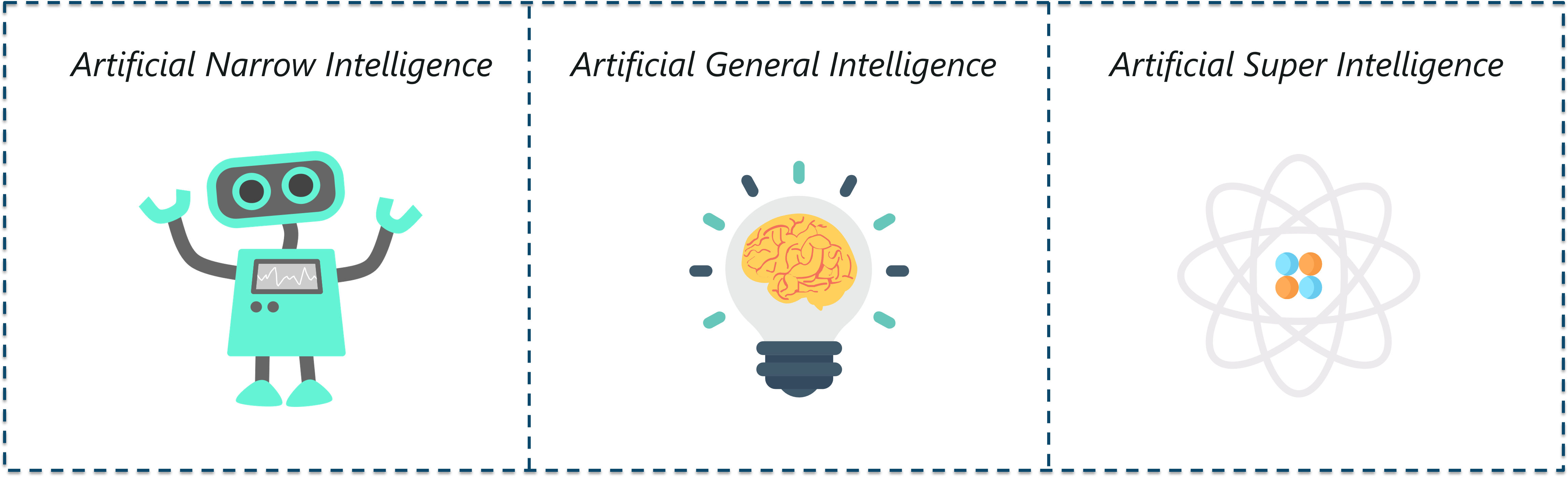Types Of AI – Artificial Intelligence With Python – Edureka

## Artificial Narrow Intelligence

Commonly known as weak AI, Artificial Narrow Intelligence involves applying AI only to specific tasks.

The existing AI-based systems that claim to use “artificial intelligence” are actually operating as a weak AI. Alexa is a good example of narrow intelligence. It operates within a limited predefined range of functions. Alexa has no genuine intelligence or self-awareness.

Google search engine, Sophia, self-driving cars and even the famous AlphaGo, fall under the category of weak AI.

## Artificial General Intelligence

Commonly known as strong AI, Artificial General Intelligence involves machines that possess the ability to perform any intellectual task that a human being can.

You see, machines don’t possess human-like abilities, they have a strong processing unit that can perform high-level computations but they’re not yet capable of thinking and reasoning like a human.

There are many experts who doubt that AGI will ever be possible, and there are also many who question whether it would be desirable.

Stephen Hawking, for example, warned:

“Strong AI would take off on its own, and re-design itself at an ever-increasing rate. Humans, who are limited by slow biological evolution, couldn’t compete and would be superseded.”

## Artificial Super Intelligence

Artificial Super Intelligence is a term referring to the time when the capability of computers will surpass humans.

ASI is presently seen as a hypothetical situation as depicted in movies and science fiction books, where machines have taken over the world. However, tech masterminds like Elon Musk believe that ASI will take over the world by 2040!

What do you think about Artificial Super Intelligence? Let me know your thoughts in the comment section.

Before I go any further, let me clear a very common misconception. I’ve been asked these question by every beginner:

What is the difference between AI and Machine Learning and Deep Learning?

## Is AI the same as ML?

Let’s break it down:

## AI vs ML vs DL (Artificial Intelligence vs Machine Learning vs Deep Learning)

People tend to think that Artificial Intelligence, Machine Learning, and Deep Learning are the same since they have common applications. For example, Siri is an application of AI, Machine learning and Deep learning.

So how are these technologies related?

• Artificial Intelligence is the science of getting machines to mimic the behavior of humans.
• Machine learning is a subset of Artificial Intelligence (AI) that focuses on getting machines to make decisions by feeding them data.
• Deep learning is a subset of Machine Learning that uses the concept of neural networks to solve complex problems.

To sum it up AI, Machine Learning and Deep Learning are interconnected fields. Machine Learning and Deep learning aids Artificial Intelligence by providing a set of algorithms and neural networks to solve data-driven problems.

However, Artificial Intelligence is not restricted to only Machine learning and Deep learning. It covers a vast domain of fields including, Natural Language Processing (NLP), object detection, computer vision, robotics, expert systems and so on.

Now let’s get started with Machine Learning.

## Machine Learning Basics

The term Machine Learning was first coined by Arthur Samuel in the year 1959. Looking back, that year was probably the most significant in terms of technological advancements.

In simple terms,

Machine learning is a subset of Artificial Intelligence (AI) which provides machines the ability to learn automatically by feeding it tons of data & allowing it to improve through experience. Thus, Machine Learning is a practice of getting Machines to solve problems by gaining the ability to think.

But how can a machine make decisions?

If you feed a machine a good amount of data, it will learn how to interpret, process and analyze this data by using Machine Learning Algorithms.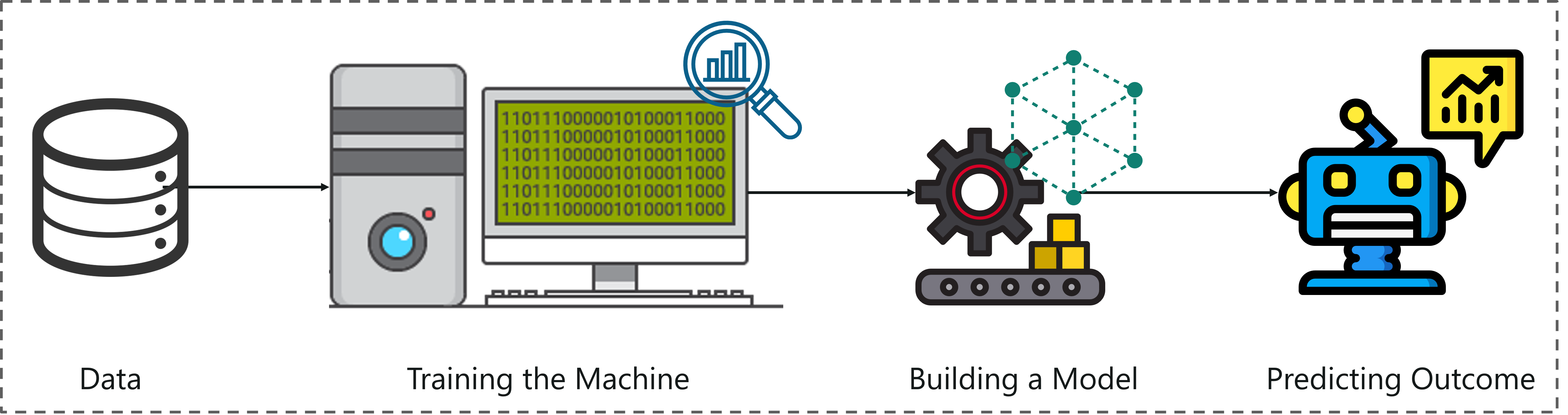What Is Machine Learning – Artificial Intelligence With Python – Edureka

To sum it up, take a look at the above figure:

• A Machine Learning process begins by feeding the machine lots of data.
• The machine is then trained on this data, to detect hidden insights and patterns.
• These insights are used to build a Machine Learning Model by using an algorithm in order to solve a problem.

Now that we know what is Machine Learning, let’s look at the different ways in which machines can learn.

## Types Of Machine Learning

A machine can learn to solve a problem by following any one of the following three approaches:

1. Supervised Learning
2. Unsupervised Learning
3. Reinforcement Learning

## Supervised Learning

Supervised learning is a technique in which we teach or train the machine using data which is well labeled.

To understand Supervised Learning let’s consider an analogy. As kids we all needed guidance to solve math problems. Our teachers helped us understand what addition is and how it is done.

Similarly, you can think of supervised learning as a type of Machine Learning that involves a guide. The labeled data set is the teacher that will train you to understand patterns in the data. The labeled data set is nothing but the training data set.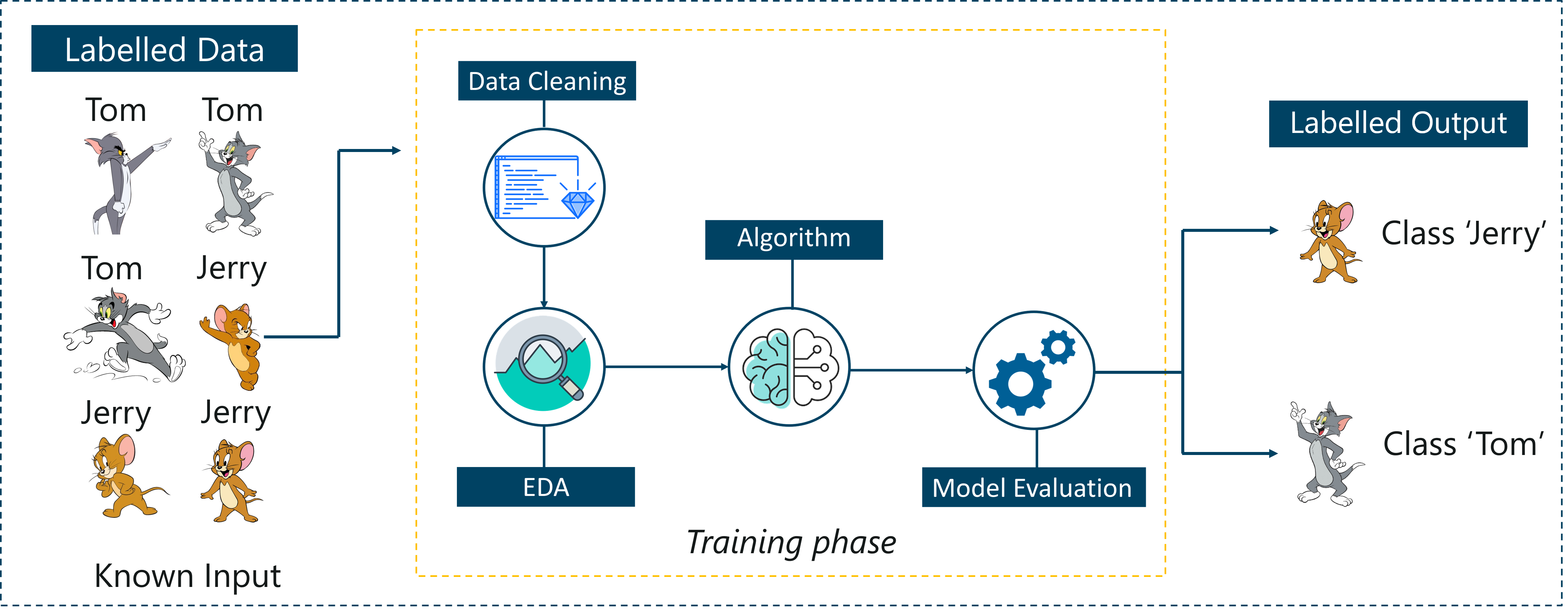Supervised Learning – Artificial Intelligence With Python – Edureka

Consider the above figure. Here we’re feeding the machine images of Tom and Jerry and the goal is for the machine to identify and classify the images into two groups (Tom images and Jerry images).

The training data set that is fed to the model is labeled, as in, we’re telling the machine, ‘this is how Tom looks and this is Jerry’. By doing so you’re training the machine by using labeled data. In Supervised Learning, there is a well-defined training phase done with the help of labeled data.

## Unsupervised Learning

Unsupervised learning involves training by using unlabeled data and allowing the model to act on that information without guidance.

Think of unsupervised learning as a smart kid that learns without any guidance. In this type of Machine Learning, the model is not fed with labeled data, as in the model has no clue that ‘this image is Tom and this is Jerry’, it figures out patterns and the differences between Tom and Jerry on its own by taking in tons of data.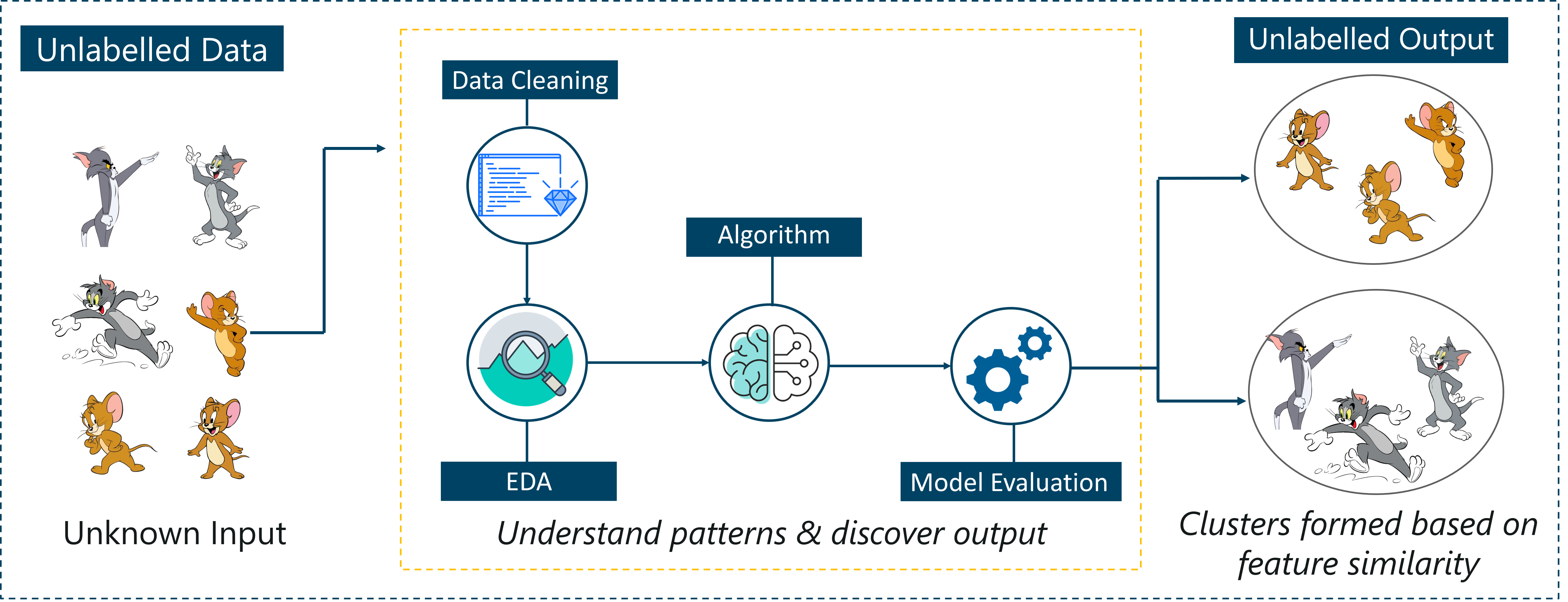Unsupervised Learning – Artificial Intelligence With Python – Edureka

For example, it identifies prominent features of Tom such as pointy ears, bigger size, etc, to understand that this image is of type 1. Similarly, it finds such features in Jerry and knows that this image is of type 2.

Therefore, it classifies the images into two different classes without knowing who Tom is or Jerry is.

## Reinforcement Learning

Reinforcement Learning is a part of Machine learning where an agent is put in an environment and he learns to behave in this environment by performing certain actions and observing the rewards which it gets from those actions.

Imagine that you were dropped off at an isolated island!

What would you do?

Panic? Yes, of course, initially we all would. But as time passes by, you will learn how to live on the island. You will explore the environment, understand the climate condition, the type of food that grows there, the dangers of the island, etc.

This is exactly how Reinforcement Learning works, it involves an Agent (you, stuck on the island) that is put in an unknown environment (island), where he must learn by observing and performing actions that result in rewards.

Reinforcement Learning is mainly used in advanced Machine Learning areas such as self-driving cars, AplhaGo, etc. So that sums up the types of Machine Learning.

Now, let’s look at the type of problems that are solved by using Machine Learning.

## What Problems Can Machine Learning Solve?

There are three main categories of problems that can be solved using Machine Learning:

### What is Regression?

In this type of problem, the output is a continuous quantity. For example, if you want to predict the speed of a car given the distance, it is a Regression problem. Regression problems can be solved by using Supervised Learning algorithms like Linear Regression.

### What is Classification?

In this type, the output is a categorical value. Classifying emails into two classes, spam and non-spam is a classification problem that can be solved by using Supervised Learning classification algorithms such as Support Vector Machines, Naive Bayes, Logistic Regression, K Nearest Neighbor, etc.

### What is Clustering?

This type of problem involves assigning the input into two or more clusters based on feature similarity. For example, clustering viewers into similar groups based on their interests, age, geography, etc can be done by using Unsupervised Learning algorithms like K-Means Clustering.### Machine Learning Course Masters Program

Here’s a table that sums up the difference between Regression, Classification, and Clustering: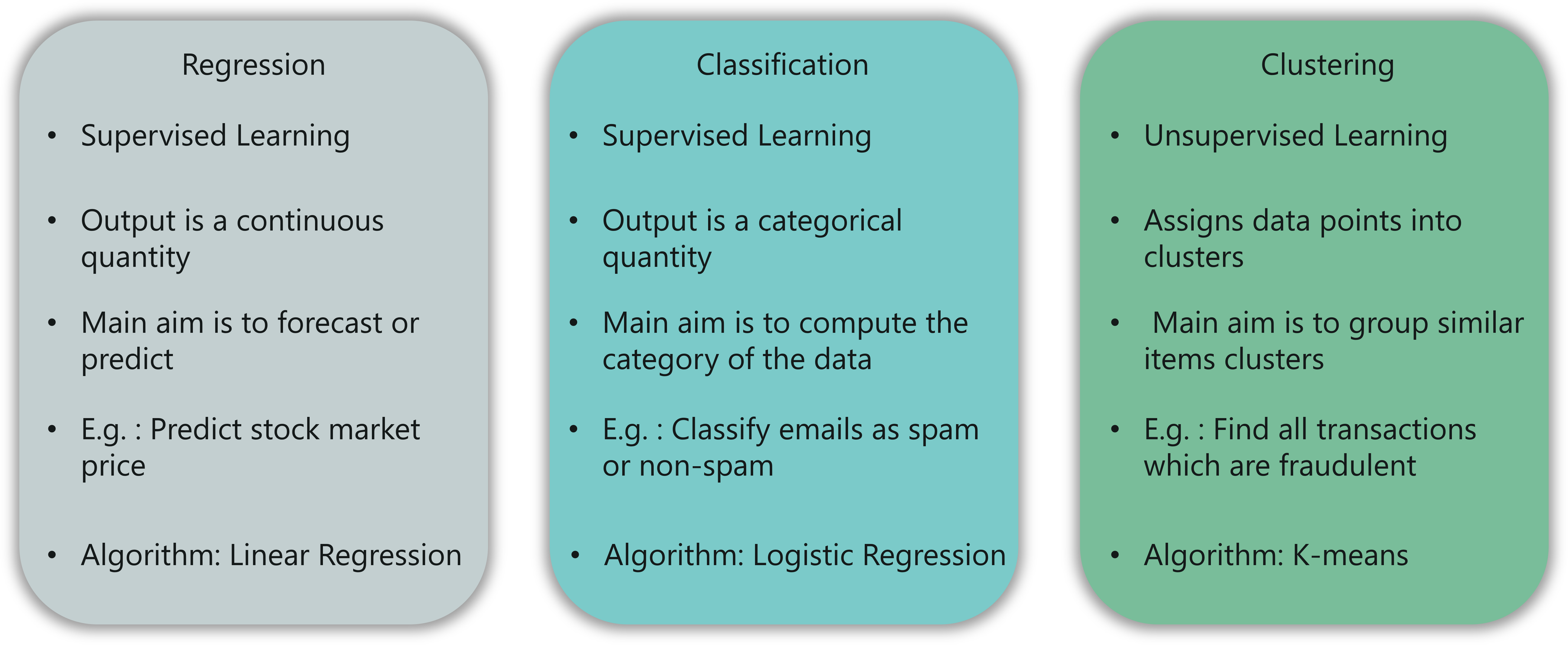Regression vs Classification vs Clustering – Artificial Intelligence With Python – Edureka

Now let’s look at how the Machine Learning process works.

## Machine Learning Process Steps

The Machine Learning process involves building a Predictive model that can be used to find a solution for a Problem Statement.

To understand the Machine Learning process let’s assume that you have been given a problem that needs to be solved by using Machine Learning.

The problem is to predict the occurrence of rain in your local area by using Machine Learning.

The below steps are followed in a Machine Learning process:

Step 1: Define the objective of the Problem Statement

At this step, we must understand what exactly needs to be predicted. In our case, the objective is to predict the possibility of rain by studying weather conditions.

It is also essential to take mental notes on what kind of data can be used to solve this problem or the type of approach you must follow to get to the solution.

Step 2: Data Gathering

At this stage, you must be asking questions such as,

• What kind of data is needed to solve this problem?
• Is the data available?
• How can I get the data?

Once you know the types of data that is required, you must understand how you can derive this data. Data collection can be done manually or by web scraping.

However, if you’re a beginner and you’re just looking to learn Machine Learning you don’t have to worry about getting the data. There are 1000s of data resources on the web, you can just download the data set and get going.

Coming back to the problem at hand, the data needed for weather forecasting includes measures such as humidity level, temperature, pressure, locality, whether or not you live in a hill station, etc.

Such data must be collected and stored for analysis.

Step 3: Data Preparation

The data you collected is almost never in the right format. You will encounter a lot of inconsistencies in the data set such as missing values, redundant variables, duplicate values, etc.

Removing such inconsistencies is very essential because they might lead to wrongful computations and predictions. Therefore, at this stage, you scan the data set for any inconsistencies and you fix them then and there.

Step 4: Exploratory Data Analysis

Grab your detective glasses because this stage is all about diving deep into data and finding all the hidden data mysteries.

EDA or Exploratory Data Analysis is the brainstorming stage of Machine Learning. Data Exploration involves understanding the patterns and trends in the data. At this stage, all the useful insights are drawn and correlations between the variables are understood.

For example, in the case of predicting rainfall, we know that there is a strong possibility of rain if the temperature has fallen low. Such correlations must be understood and mapped at this stage.

Step 5: Building a Machine Learning Model

All the insights and patterns derived during Data Exploration are used to build the Machine Learning Model. This stage always begins by splitting the data set into two parts, training data, and testing data.

The training data will be used to build and analyze the model. The logic of the model is based on the Machine Learning Algorithm that is being implemented.

In the case of predicting rainfall, since the output will be in the form of True (if it will rain tomorrow) or False (no rain tomorrow), we can use a Classification Algorithm such as Logistic Regression or Decision Tree.

Choosing the right algorithm depends on the type of problem you’re trying to solve, the data set and the level of complexity of the problem.

Step 6: Model Evaluation & Optimization

After building a model by using the training data set, it is finally time to put the model to a test.

The testing data set is used to check the efficiency of the model and how accurately it can predict the outcome.

Once the accuracy is calculated, any further improvements in the model can be implemented at this stage. Methods like parameter tuning and cross-validation can be used to improve the performance of the model.

Step 7: Predictions

Once the model is evaluated and improved, it is finally used to make predictions. The final output can be a Categorical variable (eg. True or False) or it can be a Continuous Quantity (eg. the predicted value of a stock).

In our case, for predicting the occurrence of rainfall, the output will be a categorical variable.

So that was the entire Machine Learning process.

In the next section, we will discuss the various types of Machine Learning Algorithms.

## Machine Learning Algorithms

Machine Learning Algorithms are the basic logic behind each Machine Learning model. These algorithms are based on simple concepts such as Statistics and Probability.

Follow the below-mentioned blogs to understand the Math and stats behind Machine Learning Algorithms:

1. A Complete Guide To Math And Statistics For Data Science
2. All You Need To Know About Statistics And Probability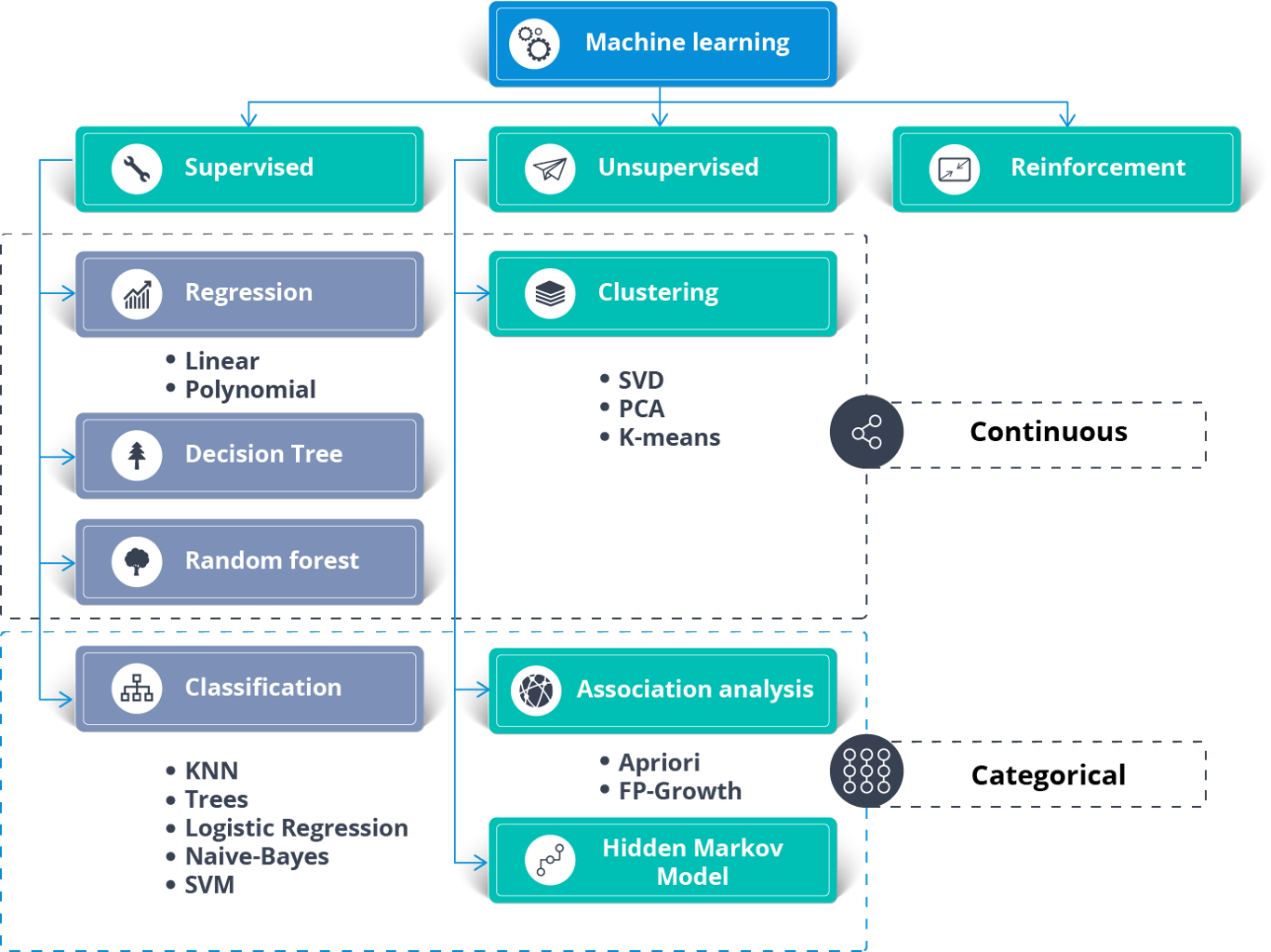Machine Learning Algorithms – Artificial Intelligence With Python – Edureka

The above figure shows the different algorithms used to solve a problem using Machine Learning.

Supervised Learning can be used to solve two types of Machine Learning problems:

1. Regression
2. Classification

To solve Regression problems you can use the famous Linear Regression Algorithm. Here’s a Linear Regression Algorithm from Scratch blog that will help you understand how it works.

Classification problems can be solved using the following Classification Algorithms:

1. Logistic Regression
2. Decision Tree
3. Random Forest
4. Naive Bayes Classifier
5. Support Vector Machine
6. K Nearest Neighbour

Unsupervised Learning can be used to solve Clustering and association problems. One of the famous clustering algorithms is the K-means Clustering algorithm.

#### Related Posts

نتیجه‌ای پیدا نشد.
فهرست